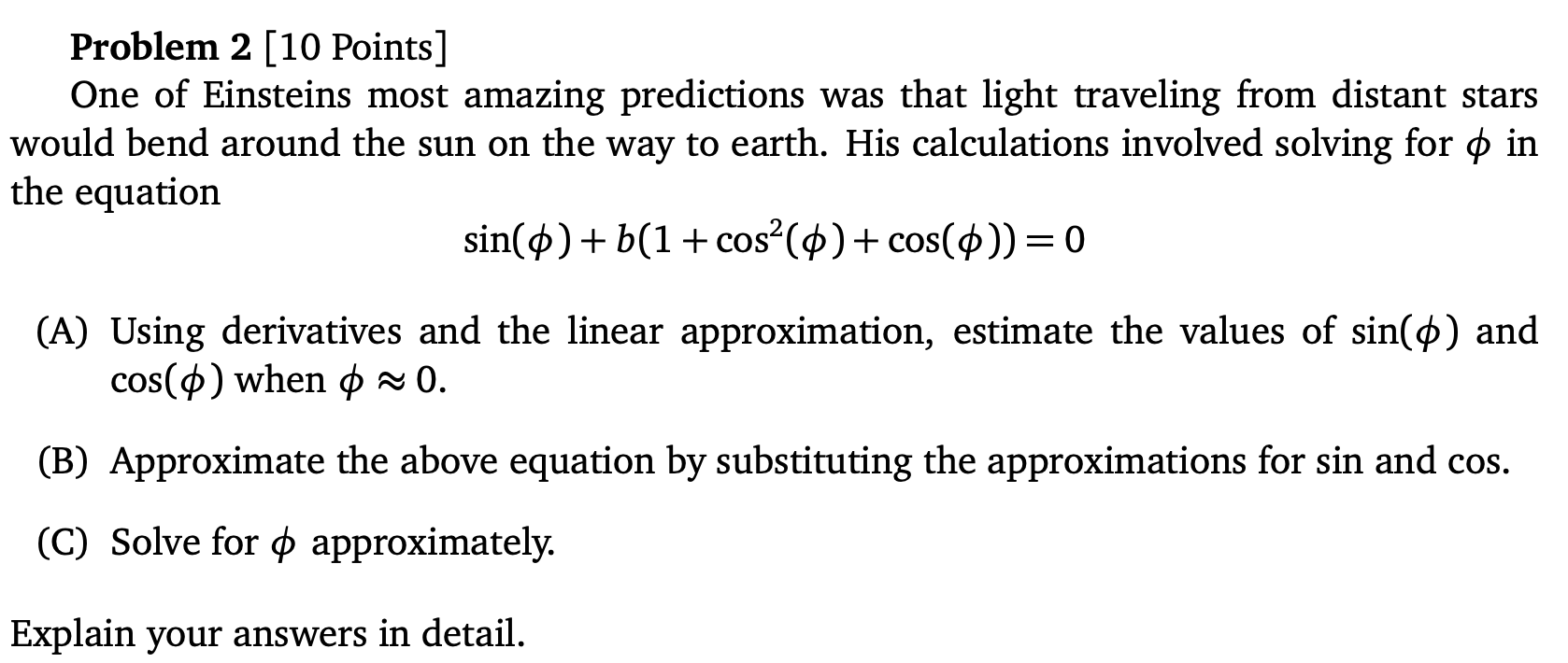# Question ​​​​​​​ Problem 2 [10 Points] One of Einsteins most amazing predictions was that light traveling from distant stars would bend around the sun on the way to earth. His calculations involved solving for $$\phi$$ in the equation $\sin (\phi)+b\left(1+\cos ^{2}(\phi)+\cos (\phi)\right)=0$ (A) Using derivatives and the linear approximation, estimate the values of $$\sin (\phi)$$ and $$\cos (\phi)$$ when $$\phi \approx 0$$. (B) Approximate the above equation by substituting the approximations for sin and cos. (C) Solve for $$\phi$$ approximately. Explain your answers in detail.​​​​​​​
Transcribed Image Text: Problem 2 [10 Points] One of Einsteins most amazing predictions was that light traveling from distant stars would bend around the sun on the way to earth. His calculations involved solving for $$\phi$$ in the equation $\sin (\phi)+b\left(1+\cos ^{2}(\phi)+\cos (\phi)\right)=0$ (A) Using derivatives and the linear approximation, estimate the values of $$\sin (\phi)$$ and $$\cos (\phi)$$ when $$\phi \approx 0$$. (B) Approximate the above equation by substituting the approximations for sin and cos. (C) Solve for $$\phi$$ approximately. Explain your answers in detail.
Transcribed Image Text: Problem 2 [10 Points] One of Einsteins most amazing predictions was that light traveling from distant stars would bend around the sun on the way to earth. His calculations involved solving for $$\phi$$ in the equation $\sin (\phi)+b\left(1+\cos ^{2}(\phi)+\cos (\phi)\right)=0$ (A) Using derivatives and the linear approximation, estimate the values of $$\sin (\phi)$$ and $$\cos (\phi)$$ when $$\phi \approx 0$$. (B) Approximate the above equation by substituting the approximations for sin and cos. (C) Solve for $$\phi$$ approximately. Explain your answers in detail.&#12304;General guidance&#12305;The answer provided below has been developed in a clear step by step manner.Step1/4Determine the derivatives of the given functions $$\mathrm{{\sin{\phi}}}$$and $$\mathrm{{\cos{\phi}}}$$as shown below:$$\mathrm{\frac{{{d}{\left\lbrace{\sin{\phi}}\right\rbrace}}}{{{d}\phi}}={\cos{\phi}}}$$$$\mathrm{\frac{{{d}{\left\lbrace{\cos{\phi}}\right\rbrace}}}{{{d}\phi}}=-{\sin{\phi}}}$$Explanation:Please refer to solution in this step.Step2/4Now evaluate the obtained derivatives at the point&#160;$$\mathrm{\phi={0}}$$.$$\mathrm{{\sin{{\left({0}\right)}}}={0}}$$$$\mathrm{{\cos{{\left({0}\right)}}}={1}}$$Therefore, the linear approximation for $$\mathrm{{\sin{\phi}}}$$is determined as follows:\begin{align*} \mathrm{{\sin{\phi}}} &\approx \mathrm{{\sin{{\left({0}\right)}}}+\frac{{{d}{\left\lbrace{\sin{\phi}}\right\rbrace}}}{{{d}\phi}}{\left(\phi-{0}\right)}}\\[3pt] &= \mathrm{{\sin{{\left({0}\right)}}}+{\cos{{\left({0}\right)}}}{\left(\phi-{0}\right)}}\\[3pt] &= \mathrm{{0}+{1}{\left(\phi-{0}\right)}}\\[3pt] &= \mathrm{\phi} \end{align*}Therefore, $$\mathrm{{\sin{\phi}}\approx\phi.}$$Explanation:Please refer to solution in this step.Step3/4Now, determin ... See the full answer